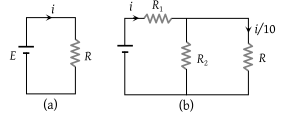NEET Questions Solved

Consider the circuits shown in the figure. Both the circuits are taking same current from battery but current through R in the second circuit is $\frac{1}{10}$th of current through R in the first circuit. If R is 11 Ω, the value of R1(1) 9.9 Ω

(2) 11 Ω

(3) 8.8 Ω

(4) 7.7 Ω

(1) In figure (b) current through ${R}_{2}=i-\frac{i}{10}=\frac{9i}{10}$

Potential difference across R2 = Potential difference across R${R}_{2}×\frac{9}{10}i=R×\frac{i}{10}$ i.e. ${R}_{2}=\frac{R}{9}=\frac{11}{9}\Omega$

${R}_{eq}=\frac{{R}_{2}×R}{\left({R}_{2}+R\right)}=\frac{\frac{11}{9}×\frac{11}{1}}{\frac{11}{9}+\frac{11}{1}}=\frac{11}{10}\Omega$

Total circuit resistance $=\frac{11}{10}+{R}_{1}=R=11$${R}_{1}=9.9\Omega$

Difficulty Level:

• 58%
• 24%
• 15%
• 5%